GATE  >  Computer Science And Information Technology - CS 2012 GATE Paper (Practice Test)

# Computer Science And Information Technology - CS 2012 GATE Paper (Practice Test) - GATE

Test Description

## 65 Questions MCQ Test GATE Past Year Papers for Practice (All Branches) - Computer Science And Information Technology - CS 2012 GATE Paper (Practice Test)

Computer Science And Information Technology - CS 2012 GATE Paper (Practice Test) for GATE 2023 is part of GATE Past Year Papers for Practice (All Branches) preparation. The Computer Science And Information Technology - CS 2012 GATE Paper (Practice Test) questions and answers have been prepared according to the GATE exam syllabus.The Computer Science And Information Technology - CS 2012 GATE Paper (Practice Test) MCQs are made for GATE 2023 Exam. Find important definitions, questions, notes, meanings, examples, exercises, MCQs and online tests for Computer Science And Information Technology - CS 2012 GATE Paper (Practice Test) below.
Solutions of Computer Science And Information Technology - CS 2012 GATE Paper (Practice Test) questions in English are available as part of our GATE Past Year Papers for Practice (All Branches) for GATE & Computer Science And Information Technology - CS 2012 GATE Paper (Practice Test) solutions in Hindi for GATE Past Year Papers for Practice (All Branches) course. Download more important topics, notes, lectures and mock test series for GATE Exam by signing up for free. Attempt Computer Science And Information Technology - CS 2012 GATE Paper (Practice Test) | 65 questions in 180 minutes | Mock test for GATE preparation | Free important questions MCQ to study GATE Past Year Papers for Practice (All Branches) for GATE Exam | Download free PDF with solutions
 1 Crore+ students have signed up on EduRev. Have you?
Computer Science And Information Technology - CS 2012 GATE Paper (Practice Test) - Question 1

### Choose the most appropriate alternative from the options given below to complete the following sentence: Despite several ––––––––– the mission succeeded in its attempt to resolve the conflict.

Computer Science And Information Technology - CS 2012 GATE Paper (Practice Test) - Question 2

### Choose the most appropriate alternative from the options given below to complete the following sentence: Suresh’s dog is the one ––––––––– was hurt in the stampede.

Computer Science And Information Technology - CS 2012 GATE Paper (Practice Test) - Question 3

### A political party orders an arch for the entrance to the ground in which the annual convention is being held. The profile of the arch follows the equation y = 2x – 0.1x2 where y is the height of the arch in meters. The maximum possible height of the arch is

Detailed Solution for Computer Science And Information Technology - CS 2012 GATE Paper (Practice Test) - Question 3

y =2x - 0.1x2
dy/dx = 2-0.2x
d2y/dx2 <0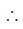y max imises at 2- 0.2x= 0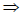x =10y= 20 - 10 =10m

Computer Science And Information Technology - CS 2012 GATE Paper (Practice Test) - Question 4

Given the sequence of terms, AD CG FK JP, the next term is _________ .

Computer Science And Information Technology - CS 2012 GATE Paper (Practice Test) - Question 5

Q. 1 – Q. 5 carry one mark each.

The cost function for a product in a firm is given by 5q2, where q is the amount of production. The
firm can sell the product at a market price of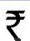50 per unit. The number of units to be produced by
the firm such that the profit is maximized is

Detailed Solution for Computer Science And Information Technology - CS 2012 GATE Paper (Practice Test) - Question 5

P = 50q - 5q2

dp/dq = 50-10q; d2p/dq2 <0p is maximum at 50- 10q = 0 or q=5

Else check with options

Computer Science And Information Technology - CS 2012 GATE Paper (Practice Test) - Question 6

Which one of the following options is the closest in meaning to the word given below?
Mitigate

Computer Science And Information Technology - CS 2012 GATE Paper (Practice Test) - Question 7

Choose the grammatically INCORRECT sentence:

Computer Science And Information Technology - CS 2012 GATE Paper (Practice Test) - Question 8

Q. 6 - Q. 10 carry two marks each.

W anted Temporary, Part-time persons for the post of Field Interviewer to conduct personal
interviews to collect and collate economic data. Requirements: High School-pass, must be
available for Day, Evening and Saturday work. Transportation paid, expenses reimbursed.

Which one of the following is the best inference from the above advertisement?

Detailed Solution for Computer Science And Information Technology - CS 2012 GATE Paper (Practice Test) - Question 8

Computer Science And Information Technology - CS 2012 GATE Paper (Practice Test) - Question 9

An automobile plant contracted to buy shock absorbers from two suppliers X and Y. X supplies 60% and Y supplies 40% of the shock absorbers. All shock absorbers are subjected to a quality test. The ones that pass the quality test are considered reliable. Of X’s shock absorbers, 96% are reliable. Of Y’s shock absorbers, 72% are reliable.

The probability that a randomly chosen shock absorber, which is found to be reliable, is made by Y is

Detailed Solution for Computer Science And Information Technology - CS 2012 GATE Paper (Practice Test) - Question 9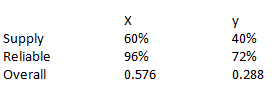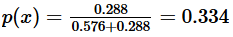Computer Science And Information Technology - CS 2012 GATE Paper (Practice Test) - Question 10

Which of the following assertions are CORRECT?
P: Adding 7 to each entry in a list adds 7 to the mean of the list
Q: Adding 7 to each entry in a list adds 7 to the standard deviation of the list
R: Doubling each entry in a list doubles the mean of the list
S: Doubling each entry in a list leaves the standard deviation of the list unchanged

Detailed Solution for Computer Science And Information Technology - CS 2012 GATE Paper (Practice Test) - Question 10

P and R always hold true
Else consider a sample set {1, 2, 3, 4} and check accordingly

Computer Science And Information Technology - CS 2012 GATE Paper (Practice Test) - Question 11

Q. 11 – Q. 35 carry one mark each.

Q.

Consider the following logical inferences.
I1: If it rains then the cricket match will not be played.
The cricket match was played.
Inference: There was no rain.
I2: If it rains then the cricket match will not be played.
It did not rain.
Inference: The cricket match was played.

Which of the following is TRUE?

Computer Science And Information Technology - CS 2012 GATE Paper (Practice Test) - Question 12

Which of the following is TRUE?

Computer Science And Information Technology - CS 2012 GATE Paper (Practice Test) - Question 13

What will be the output of the following C program segment?

char inChar = ‘A’ ;
switch ( inChar ) {
case ‘A’ : printf (“Choice A n”) ;
case ‘B’ :
case ‘C’ : printf (“Choice B”) ;
case ‘D’ :
case ‘E’ :
default : printf ( “ No Choice” ) ; }

Computer Science And Information Technology - CS 2012 GATE Paper (Practice Test) - Question 14

A ssuming P ≠ NP, which of the following is TRUE?

Computer Science And Information Technology - CS 2012 GATE Paper (Practice Test) - Question 15

T he worst case running time to search for an element in a balanced binary search tree with n2n
elements is

Computer Science And Information Technology - CS 2012 GATE Paper (Practice Test) - Question 16

The truth table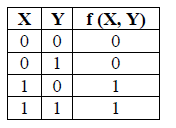Computer Science And Information Technology - CS 2012 GATE Paper (Practice Test) - Question 17

The decimal value 0.5 in IEEE single precision floating point representation has

Computer Science And Information Technology - CS 2012 GATE Paper (Practice Test) - Question 18

A process executes the code
fork();
fork();
fork();
The total number of child processes created is

Computer Science And Information Technology - CS 2012 GATE Paper (Practice Test) - Question 19

Consider the function f(x) = sin(x) in the interval x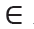[π/4, 7π/4]. The number and location(s) of the
local minima of this function are

Detailed Solution for Computer Science And Information Technology - CS 2012 GATE Paper (Practice Test) - Question 19

Sin x has a maximum value of 1 at ,
π/2 and a minimum value of –1 at

3π/2 and at all angles conterminal with them.
The graph of f (x) = sin x is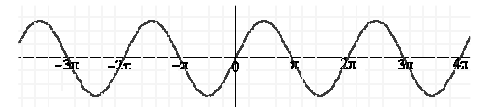In the interval   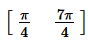, it has one local minimum x= 3π/2

Computer Science And Information Technology - CS 2012 GATE Paper (Practice Test) - Question 20

The protocol data unit (PDU) for the application layer in the Internet stack is

Detailed Solution for Computer Science And Information Technology - CS 2012 GATE Paper (Practice Test) - Question 20

The PDU for Datalink layer, Network layer , Transport layer and Application layer are frame,
datagram, segment and message respectively.

Computer Science And Information Technology - CS 2012 GATE Paper (Practice Test) - Question 21

Let A be the 2 × 2 matrix with elements a11 = a12 = a21 = +1 and a22 = −1. Then the eigenvalues of
the matrix A19 are

Detailed Solution for Computer Science And Information Technology - CS 2012 GATE Paper (Practice Test) - Question 21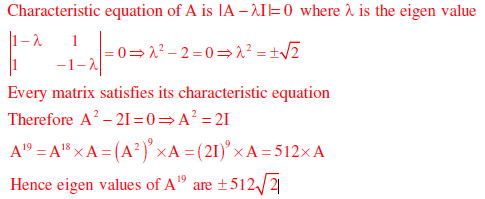Computer Science And Information Technology - CS 2012 GATE Paper (Practice Test) - Question 22

What is the complement of the language accepted by the NFA shown below?
Assume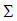= {a} and ε is the empty string.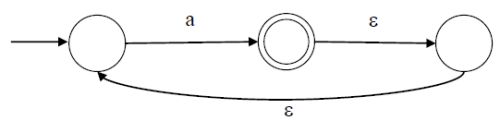Detailed Solution for Computer Science And Information Technology - CS 2012 GATE Paper (Practice Test) - Question 22

Language accepted by NFA is a+, so complement of this language is {ε}

Computer Science And Information Technology - CS 2012 GATE Paper (Practice Test) - Question 23

What is the correct translation of the following statement into mathematical logic?
“Some real numbers are rational”

Detailed Solution for Computer Science And Information Technology - CS 2012 GATE Paper (Practice Test) - Question 23

Option A: There exists x which is either real or rational and can be both.
Option B: All real numbers are rational
Option C: There exists a real number which is rational.
Option D: There exists some number which is not rational or which is real.

Computer Science And Information Technology - CS 2012 GATE Paper (Practice Test) - Question 24

Given the basic ER and relational models, which of the following is INCORRECT?

Detailed Solution for Computer Science And Information Technology - CS 2012 GATE Paper (Practice Test) - Question 24

The term ‘entity’ belongs to ER model and the term ‘relational table’ belongs to relational model.

A and B both are true. ER model supports both multivalued and composite attributes See this for more details.

(C) is false and (D) is true. In Relation model, an entry in relational table can can have exactly one value or a NULL.

Computer Science And Information Technology - CS 2012 GATE Paper (Practice Test) - Question 25

Which of the following statements are TRUE about an SQL query?
P : An SQL query can contain a HAVING clause even if it does not have a GROUP BY clause
Q : An SQL query can contain a HAVING clause only if it has a GROUP BY clause
R : All attributes used in the GROUP BY clause must appear in the SELECT clause
S : Not all attributes used in the GROUP BY clause need to appear in the SELECT clause

Detailed Solution for Computer Science And Information Technology - CS 2012 GATE Paper (Practice Test) - Question 25

If we use a HAVING clause without a GROUP BY clause, the HAVING condition applies to
all rows that satisfy the search condition. In other words, all rows that satisfy the search
condition make up a single group. So, option P is true and Q is false.
S is also true as an example consider the following table and query.0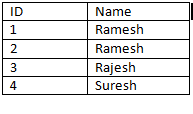Select count (*)
From student
Group by Name
Output will be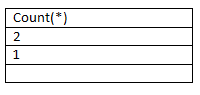Computer Science And Information Technology - CS 2012 GATE Paper (Practice Test) - Question 26

The recurrence relation capturing the optimal execution time of the Towers of Hanoi problem with
n discs is

Detailed Solution for Computer Science And Information Technology - CS 2012 GATE Paper (Practice Test) - Question 26

Let the three pegs be A,B and C, the goal is to move n pegs from A to C using peg B
The following sequence of steps are executed recursively
1.move n−1 discs from A to B. This leaves disc n alone on peg A --- T(n-1)
2.move disc n from A to C---------1
3.move n−1 discs from B to C so they sit on disc n----- T(n-1)
So, T(n) = 2T(n-1) +1

Computer Science And Information Technology - CS 2012 GATE Paper (Practice Test) - Question 27

Let G be a simple undirected planar graph on 10 vertices with 15 edges. If G is a connected graph,
then the number of bounded faces in any embedding of G on the plane is equal to

Detailed Solution for Computer Science And Information Technology - CS 2012 GATE Paper (Practice Test) - Question 27

We have the relation V-E+F=2, by this we will get the total number of faces,

F = 7. Out of 7 faces one is an unbounded face, so total 6 bounded faces.

Computer Science And Information Technology - CS 2012 GATE Paper (Practice Test) - Question 28

Let W(n) and A(n) denote respectively, the worst case and average case running time of an
algorithm executed on an input of size n. Which of the following is ALWAYS TRUE?

Detailed Solution for Computer Science And Information Technology - CS 2012 GATE Paper (Practice Test) - Question 28

The average case time can be lesser than or even equal to the worst case. So A(n) would be
upper bounded by W(n) and it will not be strict upper bound as it can even be same (e.g.
Bubble Sort and merge sort).A(n) = O(W(n))

Computer Science And Information Technology - CS 2012 GATE Paper (Practice Test) - Question 29

The amount of ROM needed to implement a 4 bit multiplier is

Detailed Solution for Computer Science And Information Technology - CS 2012 GATE Paper (Practice Test) - Question 29

For a 4 bit multiplier there are 24 × 24 = 28 = 256 combinations.
Output will contain 8 bits.
So the amount of ROM needed is  28 ×8 bits = 2Kbits.

Computer Science And Information Technology - CS 2012 GATE Paper (Practice Test) - Question 30

Register renaming is done in pipelined processors

Detailed Solution for Computer Science And Information Technology - CS 2012 GATE Paper (Practice Test) - Question 30

Register renaming is done to eliminate WAR/WAW hazards.

Computer Science And Information Technology - CS 2012 GATE Paper (Practice Test) - Question 31

Consider a random variable X that takes values +1 and −1 with probability 0.5 each. The values of
the cumulative distribution function F(x) at x = −1 and +1 are

Detailed Solution for Computer Science And Information Technology - CS 2012 GATE Paper (Practice Test) - Question 31

The cumulative distribution function

F(x) = P(X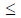x)

F(-1) = P(X-1) = X=-1) =0.5

F(+1)= P(X+1) = P(X=-1)+P(X=-1) = 0.5 + 0.5 =1

Computer Science And Information Technology - CS 2012 GATE Paper (Practice Test) - Question 32

Which of the following transport layer protocols is used to support electronic mail?

Detailed Solution for Computer Science And Information Technology - CS 2012 GATE Paper (Practice Test) - Question 32

E-mail uses SMTP, application layer protocol which intern uses TCP transport layer protocol.

Computer Science And Information Technology - CS 2012 GATE Paper (Practice Test) - Question 33

In the IPv4 addressing format, the number of networks allowed under Class C addresses is

Detailed Solution for Computer Science And Information Technology - CS 2012 GATE Paper (Practice Test) - Question 33

For class C address, size of network field is 24 bits. But first 3 bits are fixed as 110; hence
total number of networks possible is 221

Computer Science And Information Technology - CS 2012 GATE Paper (Practice Test) - Question 34

Which of the following problems are decidable?
1) Does a given program ever produce an output?
2) If L is a context-free language, then, is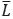also context-free?
3) If L is a regular language, then, isalso regular?
4) If L is a recursive language, then, isalso recursive?

Detailed Solution for Computer Science And Information Technology - CS 2012 GATE Paper (Practice Test) - Question 34

CFL’s are not closed under complementation. Regular and recursive languages are closed
under complementation.

Computer Science And Information Technology - CS 2012 GATE Paper (Practice Test) - Question 35

Given the language L = {ab, aa, baa}, which of the following strings are in L*?
1) abaabaaabaa
2) aaaabaaaa
3) baaaaabaaaab
4) baaaaabaa

Detailed Solution for Computer Science And Information Technology - CS 2012 GATE Paper (Practice Test) - Question 35

L ={ab, aa, baa}
Let S1 = ab , S2 = aa and S3 =baa
abaabaaabaa can be written as S1S2S3S1S2
aaaabaaaa can be written as S1S1S3S1
baaaaabaa can be written as S3S2S1S2

Computer Science And Information Technology - CS 2012 GATE Paper (Practice Test) - Question 36

Q. 36 to Q. 65 carry two marks each.

Q.

W hich of the following graphs is isomorphic to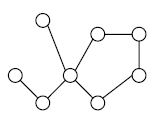Detailed Solution for Computer Science And Information Technology - CS 2012 GATE Paper (Practice Test) - Question 36

The graph in option (A) has a 3 length cycle whereas the original graph does not have a 3 length cycle
The graph in option (C) has vertex with degree 3 whereas the original graph does not have a
vertex with degree 3

The graph in option (D) has a 4 length cycle whereas the original graph does not have a 4 length cycle

Computer Science And Information Technology - CS 2012 GATE Paper (Practice Test) - Question 37

Consider the following transactions with data items P and Q initialized to zero:
if P = 0 then Q := Q + 1 ;
write (Q).
if Q = 0 then P := P + 1 ;
write (P).
Any non-serial interleaving of T1 and T2 for concurrent execution leads to

Detailed Solution for Computer Science And Information Technology - CS 2012 GATE Paper (Practice Test) - Question 37

Let S be a non-serial schedule, without loss of generality assume that T1 has started earlier than T2. The first instruction of T1 is read(P) and the last instruction of T2 is write(P), so the precedence graph for S has an edge from T1 to T2, now since S is a non-serial schedule the first instruction of T2(read(Q)) should be executed before last instruction of T1(write(Q)) and since read and write are conflicting operations, the precedence graph for S also contains an
edge from T2 to T1, So we will have a cycle in the precedence graph which implies that any non serial schedule with T1 as the earliest transaction will not be conflict serializable.
In a similar way we can show that if T2 is the earliest transaction then also the schedule is not conflict serializable.

Computer Science And Information Technology - CS 2012 GATE Paper (Practice Test) - Question 38

The bisection method is applied to compute a zero of the function f(x) = x4 – x3 – x2 – 4 in the
interval [1,9]. The method converges to a solution after ––––– iterations.

Detailed Solution for Computer Science And Information Technology - CS 2012 GATE Paper (Practice Test) - Question 38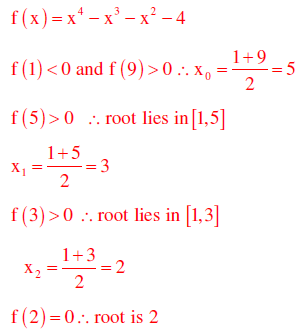Computer Science And Information Technology - CS 2012 GATE Paper (Practice Test) - Question 39

Let G be a weighted graph with edge weights greater than one and G’be the graph constructed by squaring the weights of edges in G. Let T and T’ be the minimum spanning trees of G and G’, respectively, with total weights t and t’. Which of the following statements is TRUE?

Computer Science And Information Technology - CS 2012 GATE Paper (Practice Test) - Question 40

What is the minimal form of the Karnaugh map shown below? Assume that X denotes a don’t care
term.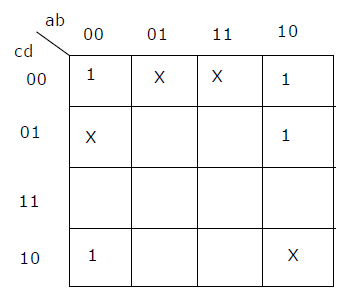Computer Science And Information Technology - CS 2012 GATE Paper (Practice Test) - Question 41

Consider the 3 processes, P1, P2 and P3 shown in the table.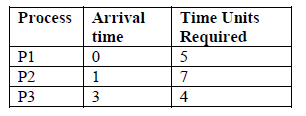The completion order of the 3 processes under the policies FCFS and RR2 (round robin scheduling
with CPU quantum of 2 time units) are

Detailed Solution for Computer Science And Information Technology - CS 2012 GATE Paper (Practice Test) - Question 41

For FCFS Execution order will be order of Arrival time so it is P1,P2,P3
Next For RR with time quantum=2,the arrangement of Ready Queue will be as follows:
RQ: P1,P2,P1,P3,P2,P1,P3,P2
This RQ itself shows the order of execution on CPU(Using Gantt Chart) and here it gives the completion order as P1,P3,P2 in Round Robin algorithm.

Computer Science And Information Technology - CS 2012 GATE Paper (Practice Test) - Question 42

memory location X, increments it by the value i, and returns the old value of X. It is used in the
pseudocode shown below to implement a busy-wait lock. L is an unsigned integer shared variable
initialized to 0. The value of 0 corresponds to lock being available, while any non-zero value
corresponds to the lock being not available.

AcquireLock(L){
L = 1;
}
ReleaseLock(L){
L = 0;
}
This implementation

Detailed Solution for Computer Science And Information Technology - CS 2012 GATE Paper (Practice Test) - Question 42

1. Acquire lock (L) {
3. L = 1.
}
4. Release Lock (L) {
5. L = 0;
6. }
Let P and Q be two concurrent processes in the system currently executing as follows
P executes 1,2,3 then Q executes 1 and 2 then P executes 4,5,6 then L=0 now Q executes 3
by which L will be set to 1 and thereafter no process can set
L to zero, by which all the processes could starve.

Computer Science And Information Technology - CS 2012 GATE Paper (Practice Test) - Question 43

Suppose a fair six-sided die is rolled once. If the value on the die is 1, 2, or 3, the die is rolled a
second time. What is the probability that the sum total of values that turn up is at least 6?

Computer Science And Information Technology - CS 2012 GATE Paper (Practice Test) - Question 44

An Internet Service Provider (ISP) has the following chunk of CIDR-based IP addresses available
with it: 245.248.128.0/20. The ISP wants to give half of this chunk of addresses to Organization A,
and a quarter to Organization B, while retaining the remaining with itself. Which of the following is
a valid allocation of addresses to A and B?

Detailed Solution for Computer Science And Information Technology - CS 2012 GATE Paper (Practice Test) - Question 44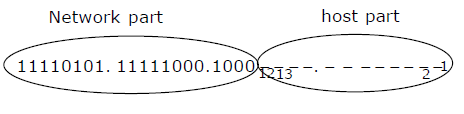Since half of 4096 host addresses must be given to organization A, we can set 12th bit to 1 and include that bit into network part of organization A, so the valid allocation of addresses to A is 245.248.136.0/21
Now for organization B, 12th bit is set to ‘0’ but since we need only half of 2048 addresses,
13th bit can be set to ‘0’ and include that bit into network part of organization B so the valid allocation of addresses to B is 245.248.128.0/22

Computer Science And Information Technology - CS 2012 GATE Paper (Practice Test) - Question 45

Suppose a circular queue of capacity (n −1) elements is implemented with an array of n elements.
Assume that the insertion and deletion operations are carried out using REAR and FRONT as array
index variables, respectively. Initially, REAR = FRONT = 0. The conditions to detect queue full
and queue empty are

Detailed Solution for Computer Science And Information Technology - CS 2012 GATE Paper (Practice Test) - Question 45

The counter example for the condition full : REAR = FRONT is
Initially when the Queue is empty REAR=FRONT=0 by which the above full condition is satisfied which is false
The counter example for the condition full : (FRONT+1)mod n =REAR is
Initially when the Queue is empty REAR=FRONT=0 and let n=3, so after inserting one element REAR=1 and FRONT=0, at this point the condition full above is satisfied, but still there is place for one more element in Queue, so this condition is also false
The counter example for the condition empty : (REAR+1)mod n = FRONT is
Initially when the Queue is empty REAR=FRONT=0 and let n=2, so after inserting one element REAR=1 and FRONT=0, at this point the condition empty above is satisfied, but the queue of capacity n-1 is full here
The counter example for the condition empty : (FRONT+1)mod n =REAR is
Initially when the Queue is empty REAR=FRONT=0 and let n=2, so after inserting one element REAR=1 and FRONT=0, at this point the condition empty above is satisfied, but the queue of capacity n-1 is full here

Computer Science And Information Technology - CS 2012 GATE Paper (Practice Test) - Question 46

Consider the program given below, in a block-structured pseudo-language with lexical scoping and
nesting of procedures permitted.

Program main;
Var ...
Procedure A1;
Var ...
Call A2;
End A1
Procedure A2;
Var ...
Procedure A21;
Var ...
Call A1;
End A21

Call A21;
End A2
Call A1;
End main.
Consider the calling chain: Main → A1 → A2 → A21 → A1
The correct set of activation records along with their access links is given by

Detailed Solution for Computer Science And Information Technology - CS 2012 GATE Paper (Practice Test) - Question 46

Access link is defined as link to activation record of closest lexically enclosing block in
program text, so the closest enclosing blocks respectively for A1 ,A2 and A21 are main , main and A2

Computer Science And Information Technology - CS 2012 GATE Paper (Practice Test) - Question 47

How many onto (or surjective) functions are there from an n-element (n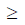2) set to a 2-element set?

Detailed Solution for Computer Science And Information Technology - CS 2012 GATE Paper (Practice Test) - Question 47

Total number of functions is 2n, out of which there will be exactly two functions where all
elements map to exactly one element, so total number of onto functions is 2n-2

Computer Science And Information Technology - CS 2012 GATE Paper (Practice Test) - Question 48

Let G be a complete undirected graph on 6 vertices. If vertices of G are labeled, then the number of
distinct cycles of length 4 in G is equal to

Computer Science And Information Technology - CS 2012 GATE Paper (Practice Test) - Question 49

A list of n strings, each of length n, is sorted into lexicographic order using the merge-sort
algorithm. The worst case running time of this computation is

Detailed Solution for Computer Science And Information Technology - CS 2012 GATE Paper (Practice Test) - Question 49

The height of the recursion tree using merge sort is logn and n2 comparisons are done at each
level, where at most n pairs of strings are compared at each level and n comparisons are
required to compare any two strings, So the worst case running time is O( n2 log n )

Computer Science And Information Technology - CS 2012 GATE Paper (Practice Test) - Question 50

Consider the directed graph shown in the figure below. There are multiple shortest paths between
vertices S and T. Which one will be reported by Dijkstra’s shortest path algorithm? Assume that, in
any iteration, the shortest path to a vertex v is updated only when a strictly shorter path to v is
discovered.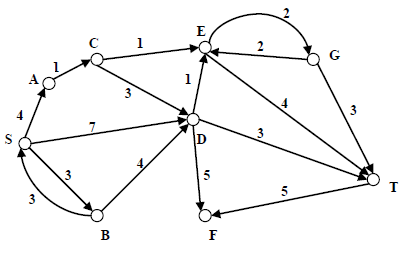Detailed Solution for Computer Science And Information Technology - CS 2012 GATE Paper (Practice Test) - Question 50

Let d[v] represent the shortest path distance computed from ‘S’
Initially d[S]=0, d[A] = ∞, d[B] = ∞.− − − −,d[T] = ∞
And let P[v] represent the predecessor of v in the shortest path from ‘S’ to ‘v’ and let P[v]=-1
denote that currently predecessor of ‘v’ has not been computed
→ Let Q be the set of vertices for which shortest path distance has not been computed
→ Let W be the set of vertices for which shortest path distance has not been computed
→ So initially, Q = {S,A,B,C,D,E,F,G,T},W = f
We will use the following procedure
Repeat until Q is empty
{
1 u = choose a vertex from Q with minimum d[u] value
2. Q = Q − u
3. update all the adjacent vertices of u
4. W = W U{u}
}

d[S] = 0, d[A] = ∞, d[B] = ∞ ,………, d[T] = ∞

Step 1 : u = S
Step 2 : Q ={A,B,C,D,E,F,G,T}
Step 3: final values after adjustment
d[S] = 0,d[A] = 4, d[B] = 3,d[C] = ∞,d[D] = 7,d[E] = ∞ − −−,d[T] = ∞
P[A] = S, P[B] = S,P[C] = −1,P[D] = S,P[E] = −1− −−,P[T] = −1
Step 4 : W={S}

Iteration 2:
Step 1: u= B
Step 2 :Q ={A,C,D,E,F,G,T}
step 3: final values after adjustment
d[S] = 0,d[A] = 4, d[B] = 3,d[C] = ∞,d[D] = 7,d[E] = ∞ − −−,d[T] = ∞
P[A] = S, P[B] = S,P[C] = −1,P[D] = S,P[E] = −1− −−,P[T] = −1
Step 4 : W={S,B}

Iteration 3:
Step 1: u= A
Step 2 :Q ={C,D,E,F,G,T}
step 3: final values after adjustment
d[S] = 0,d[A] = 4, d[B] = 3,d[C] = 5,d[D] = 7,d[E] = ∞ − −−,d[T] = ∞
P[A] = S, P[B] = S,P[C] = A,P[D] = S,P[E] = −1− −−,P[T] = −1
Step 4 : W={S,B,A}

Iteration 4:
Step 1: u= A
Step 2 :Q ={D,E,F,G,T}
step 3: final values after adjustment
d[S] = 0,d[A] = 4, d[B] = 3,d[C] = 5,d[D] = 7,d[E] = 6 − −−,d[T] = ∞
P[A] = S, P[B] = S,P[C] = A,P[D] = S,P[E] = −1− −−,P[T] = −1
Step 4 : W={S,B,A,C}

Iteration 5:
Step 1: u= E
Step 2 :Q ={D,F,G,T}
step 3: final values after adjustment
d[S] = 0,d[A] = 4, d[B] = 3,d[C] = 5,d[D] = 7,d[E] = 6,d[F] = ∞,d[G] = 8,d[T] = 10
P[A] = S, P[B] = S,P[C] = A,,P[D] = S,P[E] =C, P[F]= −1, P[G]=E, P[T] = E
Step 4 : W={S,B,A,C,E}

After iteration 5, we can observe that P[T]=E , P[E]=C , P[C]=A , P[A]=S,
So the shortest path from S to T is SACET

Computer Science And Information Technology - CS 2012 GATE Paper (Practice Test) - Question 51

A file system with 300 GByte disk uses a file descriptor with 8 direct block addresses, 1 indirect
block address and 1 doubly indirect block address. The size of each disk block is 128 Bytes and the
size of each disk block address is 8 Bytes. The maximum possible file size in this file system is

Detailed Solution for Computer Science And Information Technology - CS 2012 GATE Paper (Practice Test) - Question 51

Each block size = 128 Bytes
Disk block address = 8 Bytes
Each disk can contain =
128
16
8
Size due to 8 direct block addresses: 8 x 128
Size due to 1 indirect block address: 16 x 128
Size due to 1 doubly indirect block address: 16 x 16 x 128
Size due to 1 doubly indirect block address: 16 x 16 x 128

Computer Science And Information Technology - CS 2012 GATE Paper (Practice Test) - Question 52

Consider the virtual page reference string
1, 2, 3, 2, 4, 1, 3, 2, 4, 1
on a demand paged virtual memory system running on a computer system that has main memory
size of 3 page frames which are initially empty. Let LRU, FIFO and OPTIMAL denote the number
of page faults under the corresponding page replacement policy. Then

Detailed Solution for Computer Science And Information Technology - CS 2012 GATE Paper (Practice Test) - Question 52

FIFO
1 1 1 4 4 4
2 2 2 1 1
3 3 3 2                           → (6) faults
Optimal
1 1 1 1 1
2 2 4 4
3 3 2                               → (5) faults
LRU
1 1 1 4 4 4 2 2 2
2 2 2 2 3 3 3 1
3 3 1 1 1 4 4                    → (9) faults
Optimal < FIFO < LRU

Computer Science And Information Technology - CS 2012 GATE Paper (Practice Test) - Question 53

Suppose R1(A, B) and R2(C, D) are two relation schemas. Let r1 and r2 be the corresponding
relation instances. B is a foreign key that refers to C in R2. If data in r1 and r2 satisfy referential
integrity constraints, which of the following is ALWAYS TRUE?

Detailed Solution for Computer Science And Information Technology - CS 2012 GATE Paper (Practice Test) - Question 53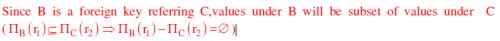Computer Science And Information Technology - CS 2012 GATE Paper (Practice Test) - Question 54

Consider a source computer (S) transmitting a file of size 106 bits to a destination computer (D)
over a network of two routers (R1 and R2) and three links (L1, L2, and L3). L1 connects S to R1; L2
connects R1 to R2; and L3 connects R2 to D. Let each link be of length 100 km. Assume signals
travel over each link at a speed of 108 meters per second. Assume that the link bandwidth on each
link is 1Mbps. Let the file be broken down into 1000 packets each of size 1000 bits. Find the total
sum of transmission and propagation delays in transmitting the file from S to D?

Detailed Solution for Computer Science And Information Technology - CS 2012 GATE Paper (Practice Test) - Question 54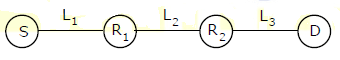Transmission delay for 1 packet from each of S, R1 and R2 will take 1ms
Propagation delay on each link L1, L2 and L3 for one packet is 1ms
Therefore the sum of transmission delay and propagation delay on each link for one packet is
2ms.
The first packet reaches the destination at 6thms
The second packet reaches the destination at 7thms
So inductively we can say that 1000th packet reaches the destination at 1005th ms

Computer Science And Information Technology - CS 2012 GATE Paper (Practice Test) - Question 55

Consider an instance of TCP’s Additive Increase Multiplicative Decrease (AIMD) algorithm where
the window size at the start of the slow start phase is 2 MSS and the threshold at the start of the first
transmission is 8 MSS. Assume that a timeout occurs during the fifth transmission. Find the
congestion window size at the end of the tenth transmission.

Computer Science And Information Technology - CS 2012 GATE Paper (Practice Test) - Question 56

Consider the set of strings on {0,1} in which, every substring of 3 symbols has at most two zeros.
For example, 001110 and 011001 are in the language, but 100010 is not. All strings of length less
than 3 are also in the language. A partially completed DFA that accepts this language is shown
below.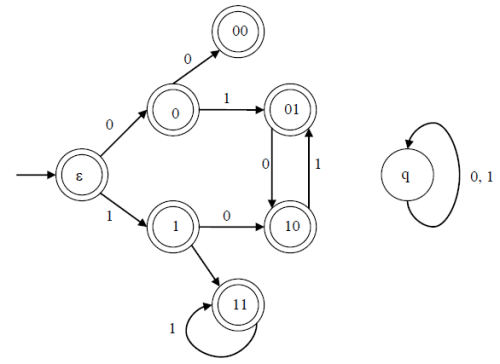The missing arcs in the DFA are

Computer Science And Information Technology - CS 2012 GATE Paper (Practice Test) - Question 57

The height of a tree is defined as the number of edges on the longest path in the tree. The function
shown in the pseudocode below is invoked as height(root) to compute the height of a binary
tree rooted at the tree pointer root.
int height (treeptr n)
{ if (n == NULL) return -1;
if (n → left == NULL)
if (n → right == NULL) return 0;
else return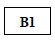;  // Box 1
else { h1 = height (n → left);
if (n → right == NULL) return (1+h1);
else { h2 = height (n → right);
return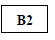; // Box 2
}
}
}
The appropriate expressions for the two boxes B1 and B2 are

Detailed Solution for Computer Science And Information Technology - CS 2012 GATE Paper (Practice Test) - Question 57

int height (treeptr n)
{ if (n = = nu11) return – 1;
→/* If there is no node, return -1 */
if (n→left ==NULL) ® / * If there is no left child for node 'n ' * /
if (n→right == NULL) return O;
→ / *If no left child & no right child for 'n ', return */
else return (1+height (n→right ) );
→/* If no left child, but there is a right child, then compute height
for right sub tree. Therefore total height is 1+ height (n→right ) */
else { → / * If there exist left child node for node ‘n’ */
h1 = height (n→left );
→ / * First Find the height of left sub tree for node ‘n’ */
If (n → right == NULL) return (1+ h1);
|CS-GATE-2012 PAPER|
 India’s No.1 institute for GATE Training  1 Lakh+ Students trained till date  65+ Centers across India
11
→/ * If there exist left child and no right child and no right child
for a node ‘n’, then total height
= height from (n to n→left ) + left sub tree height
=1 + height (n →left ) = 1 + h1 */
else {h2 = height (n →right ) ;
→/* If there exist right child also, then compute height of right
sub tree for a node ‘n’ */
return ( ( )) 1 2 1+ max h , h ;
→ / * Total height for node ‘n’=
1 + Max (Left Subtree height, Right sub tree height)
= 1 + Max (h1, h2) */
}
}

Computer Science And Information Technology - CS 2012 GATE Paper (Practice Test) - Question 58

Consider the following C code segment.
int a, b, c = 0;
void prtFun(void);
main( )
{ static int a = 1; /* Line 1 */
prtFun( );
a += 1;
prtFun( );
printf(“ %d %d ”, a, b);
}
void prtFun(void)
{ static int a = 2; /* Line 2 */
int b = 1;
a += ++b;
printf(“ %d %d ”, a, b);
}

What output will be generated by the given code segment?

Computer Science And Information Technology - CS 2012 GATE Paper (Practice Test) - Question 59

Consider the following C code segment.
int a, b, c = 0;
void prtFun(void);
main( )
{ static int a = 1; /* Line 1 */
prtFun( );
a += 1;
prtFun( );
printf(“ %d %d ”, a, b);
}
void prtFun(void)
{ static int a = 2; /* Line 2 */
int b = 1;
a += ++b;
printf(“ %d %d ”, a, b);
}

What output will be generated by the given code segment if:
Line 1 is replaced by auto int a = 1;
Line 2 is replaced by register int a = 2;

Computer Science And Information Technology - CS 2012 GATE Paper (Practice Test) - Question 60

Consider the following relations A, B and C: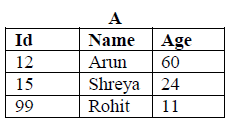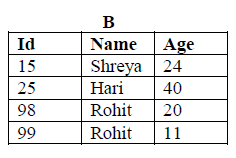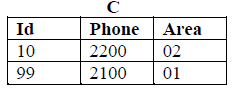How many tuples does the result of the following relational algebra expression contain? Assume
that the schema of A∪B is the same as that of A.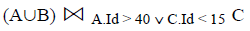Computer Science And Information Technology - CS 2012 GATE Paper (Practice Test) - Question 61

Consider the following relations A, B and C:How many tuples does the result of the following SQL query contain?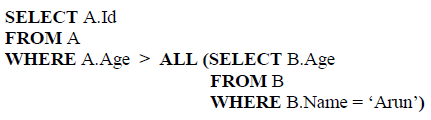Computer Science And Information Technology - CS 2012 GATE Paper (Practice Test) - Question 62

For the grammar below, a partial LL(1) parsing table is also presented along with the grammar. Entries that
need to be filled are indicated as E1, E2, and E3. ε is the empty string, \$ indicates end of input, and, |
separates alternate right hand sides of productions.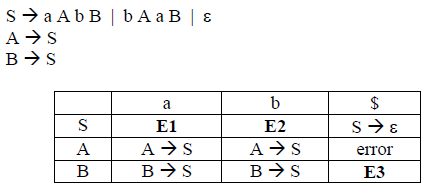The FIRST and FOLLOW sets for the non-terminals A and B are

Detailed Solution for Computer Science And Information Technology - CS 2012 GATE Paper (Practice Test) - Question 62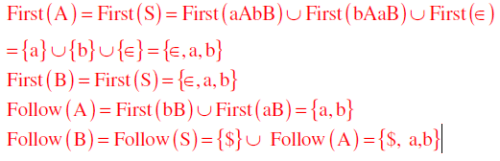Computer Science And Information Technology - CS 2012 GATE Paper (Practice Test) - Question 63

For the grammar below, a partial LL(1) parsing table is also presented along with the grammar. Entries that
need to be filled are indicated as E1, E2, and E3. ε is the empty string, \$ indicates end of input, and, |
separates alternate right hand sides of productions.The appropriate entries for E1, E2, and E3 are

Computer Science And Information Technology - CS 2012 GATE Paper (Practice Test) - Question 64

A computer has a 256 KByte, 4-way set associative, write back data cache with block size of 32 Bytes. The
processor sends 32 bit addresses to the cache controller. Each cache tag directory entry contains, in
addition to address tag, 2 valid bits, 1 modified bit and 1 replacement bit.

The number of bits in the tag field of an address is

Computer Science And Information Technology - CS 2012 GATE Paper (Practice Test) - Question 65

A computer has a 256 KByte, 4-way set associative, write back data cache with block size of 32 Bytes. The
processor sends 32 bit addresses to the cache controller. Each cache tag directory entry contains, in
addition to address tag, 2 valid bits, 1 modified bit and 1 replacement bit.

The size of the cache tag directory is

## GATE Past Year Papers for Practice (All Branches)

407 docs|127 tests
Information about Computer Science And Information Technology - CS 2012 GATE Paper (Practice Test) Page
In this test you can find the Exam questions for Computer Science And Information Technology - CS 2012 GATE Paper (Practice Test) solved & explained in the simplest way possible. Besides giving Questions and answers for Computer Science And Information Technology - CS 2012 GATE Paper (Practice Test), EduRev gives you an ample number of Online tests for practice

## GATE Past Year Papers for Practice (All Branches)

407 docs|127 tests